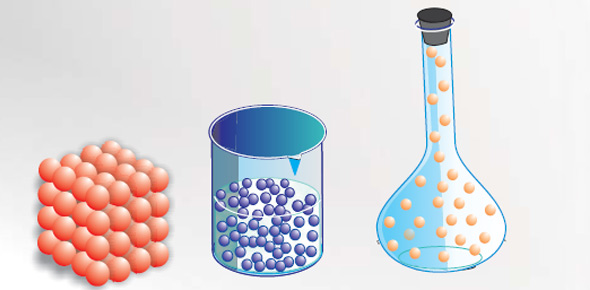# Chapter 2: The Properties Of Matter

Approved & Edited by ProProfs Editorial Team
At ProProfs Quizzes, our dedicated in-house team of experts takes pride in their work. With a sharp eye for detail, they meticulously review each quiz. This ensures that every quiz, taken by over 100 million users, meets our standards of accuracy, clarity, and engagement.
| Written by Wiebnerc
W
Wiebnerc
Community Contributor
Quizzes Created: 1 | Total Attempts: 116
Questions: 9 | Attempts: 119SettingsComplete this quiz as a review for the Chapter 2 test on the Properties of Matter! In addition to knowing this information, please make sure that you are able to demonstrate how to use the balance and beaker to measure mass and volume of various objects and liquids.

• 1.

### The mass of an object can change if:

• A.

The object is moved to a different height.

• B.

The object is put under water.

• C.

The object is moved to a place where the gravity is different.

• D.

None of the above

D. None of the above
Explanation
The mass of an object does not change under normal circumstances.

Rate this question:

• 2.

### A balance can measure the mass of:

• A.

Solids

• B.

Liquids

• C.

Solids and liquids

• D.

Only very heavy things

C. Solids and liquids
Explanation
A balance is a measuring instrument used to determine the mass of an object. It works based on the principle of comparing the weight of the object to a known weight. Solids and liquids both have mass, and therefore can be measured using a balance. This is because mass is a fundamental property of matter and is independent of the state of the substance. Therefore, the correct answer is that a balance can measure the mass of both solids and liquids.

Rate this question:

• 3.

### If you place a marble in a half-filled glass of water, the water level will:

• A.

Rise

• B.

Fall

• C.

Stay the same

• D.

Overflow

A. Rise
Explanation
When a marble is placed in a half-filled glass of water, the water level will rise. This is because the marble displaces a certain volume of water equal to its own volume. As a result, the water level increases to accommodate the added volume of the marble.

Rate this question:

• 4.

### To find the density of a substance, you must know:

• A.

• B.

How much it weighs

• C.

Its mass and volume

• D.

Its length and width

C. Its mass and volume
Explanation
To find the density of a substance, you need to know its mass and volume. Density is defined as the mass of a substance per unit volume. By dividing the mass of the substance by its volume, you can determine its density. Knowing what the substance is made of, how much it weighs, or its length and width are not directly related to finding its density. Mass and volume are the essential parameters required to calculate density accurately.

Rate this question:

• 5.

### What is used with a balance to measure and object's mass?

• A.

Paper clips

• B.

Scale

• C.

Standard mass

• D.

C. Standard mass
Explanation
A standard mass is used with a balance to measure an object's mass. A balance works by comparing the mass of the object being measured with the mass of known standard masses. By placing the object on one side of the balance and the standard mass on the other side, the balance can determine the mass of the object based on how it balances out. Therefore, the correct answer is standard mass.

Rate this question:

• 6.

### What is the metric unit of weight?

• A.

Gram

• B.

Newton

• C.

Pound

• D.

Milliliter

B. Newton
Explanation
The gram is the metric unit for MASS... not weight.

Rate this question:

• 7.

### What has a weight of 9.8 Newtons?

• A.

A 50-gram standard mass

• B.

A hundred-pound box

• C.

• D.

A one-kilogram mass

D. A one-kilogram mass
Explanation
A one-kilogram mass has a weight of 9.8 Newtons because weight is the force exerted on an object due to gravity. The weight of an object can be calculated by multiplying its mass by the acceleration due to gravity, which is approximately 9.8 meters per second squared. Therefore, a one-kilogram mass will have a weight of 9.8 Newtons.

Rate this question:

• 8.

### To find _____________, divide the mass by the volume.

density
the density
Explanation
To find the density, you need to divide the mass by the volume. Density is a measure of how much mass is contained within a given volume. By dividing the mass by the volume, you can determine how much mass is present in a specific amount of space, which is the density.

Rate this question:

• 9.

### Matter with a density that is greater than water will ___________ when placed in a container of water.Back to top